# Subtraction with Decimals

Times up!
Times up! You may finish answering the current question and then proceed to your score!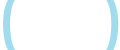Sorry, you have used all of your available hints for this lesson.
Practice Limit Reached

You've reached your daily practice limit of 12 questions.

Award InformationAwarded toQuestion HintChoose Family Member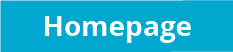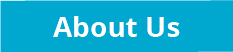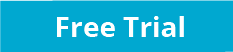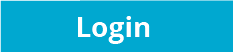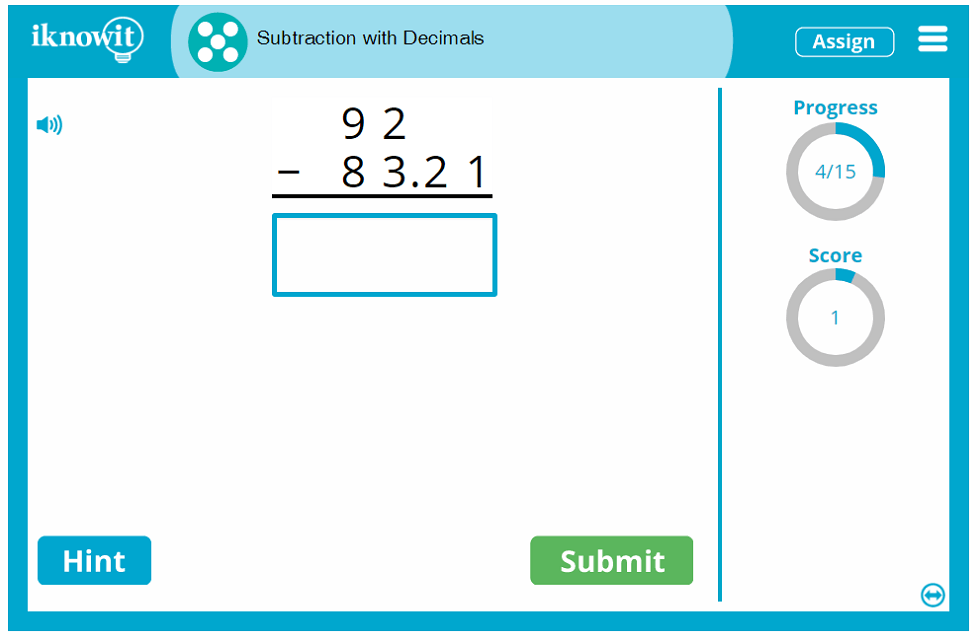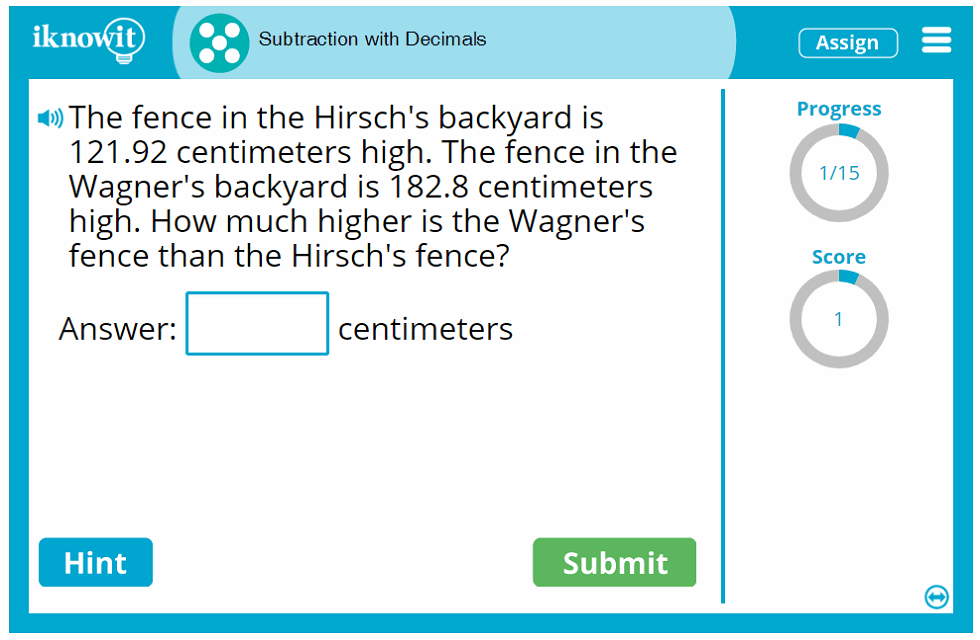## Interactive Math Lesson - Subtraction with Decimals

Students will practice subtraction with decimals up to the thousandths place in this fun, online math activity geared toward fifth grade. Put those subtraction skills to the test with decimal subtraction that challenges students to line up the decimals and subtract the fractional parts. This math activity will help fifth grade students sharpen their decimal subtraction skills and gain confidence with decimal math problems.

In this interactive math game, students will solve a variety of decimal subtraction problems. Some math problems are presented as vertical subtraction problems, while others are presented as horizontal subtraction problems. Kids may even be asked several subtraction word problems, like this one: "Gianna bought a bag of candy that weighed 47.9 grams. She shared the candy with her friends during lunch. Altogether they ate 28.349 grams of candy. How many grams of candy are left?" If students need a little extra help solving a math problem, they can click on the "Hint" button in the bottom-left corner of their practice screen. They will be shown a helpful picture that will get them started with the first step, "Line up the decimals," and the second step, "Subtract the fractional parts." When students answer a subtraction problem incorrectly, a detailed explanation page will display the correct answer, accompanied by an easy-to-follow guide to obtaining the right answer, step-by-step. Students will move through the subtraction activity having learned from past mistakes.

This fifth-grade subtraction game is equipped with several helpful features that assist children with their math practice. A progress-tracker in the upper-right corner of the practice screen shows students how many questions they have answered out of the total number of questions in the math lesson. A score-tracker lets students know how many points they have earned so far for solving math problems correctly. Opposite, the speaker icon indicates the handy read-aloud feature; students can click this button to have the question read out loud to them in a clear voice. This option is ideal for ESL/ELL students and children who excel with auditory learning. All of these features are designed with students' math practice success in mind.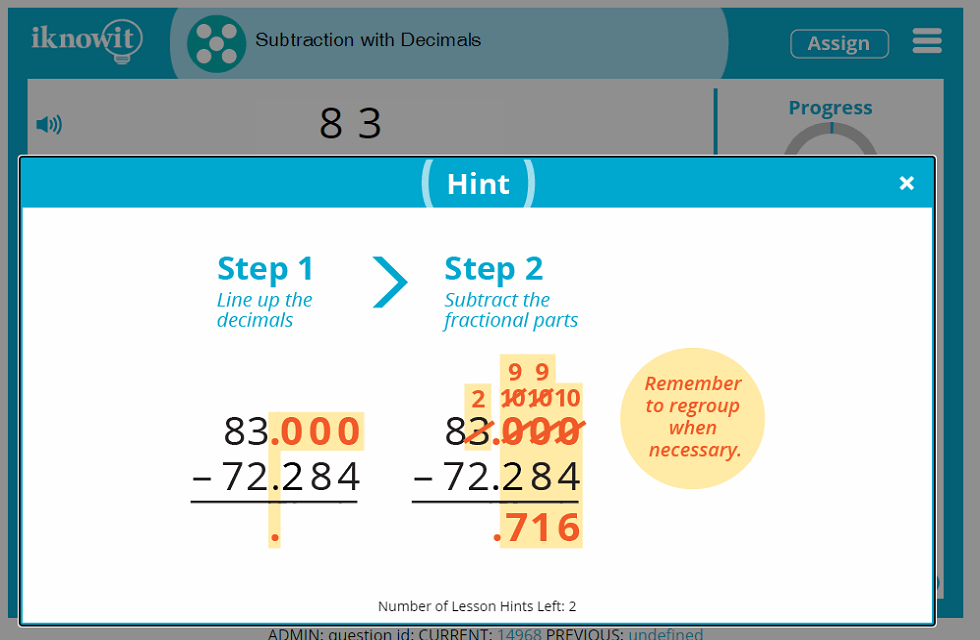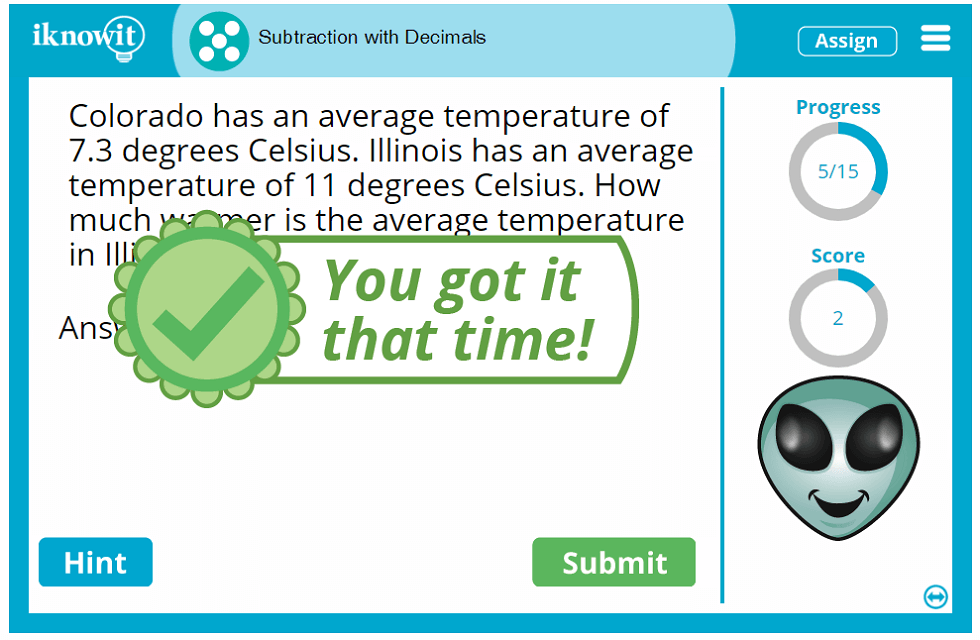## 'I Know It' Is Preferred by Educators and Students

Elementary teachers, homeschool educators, and students alike love using the "I Know It" math program to practice math skills in the classroom and at home. Educators appreciate the wide variety of math topics we cover with our interactive math games ranging from kindergarten to fifth grade. Every math activity on our website is thoughtfully written to meet at least one Common Core Standard. All math games are arranged on the website by grade level and topic, making it easy to find and assign practice activities with just a few clicks. Students, too, enjoy practicing math skills using the "I Know It" online platform. Learning is fun with cute animated characters, math trophies for new skills learned, and plenty of kid-friendly positive reinforcement. Math practice with "I Know It" is a win for everyone!

We hope you and your students will try out this fifth-grade decimal subtraction activity and discover all there is to love about "I Know It"! Be sure to check out our other fifth grade math topics too!

Sign up today for a free thirty-day trial of the "I Know It" program. You can try out this fifth-grade math game, as well as any math activity on our website, at no cost for a full thirty days. We're confident you and your students will love the difference interactive math practice can make; when your free trial runs out, we hope you will consider joining the "I Know It" community so you can continue to enjoy the benefits of interactive math practice for a full calendar year! We offer convenient membership options for families, individual teachers, schools, and school districts. Our friendly sales team would be happy to answer any questions you may have about "I Know It" membership - email Andy (andy@iknowit.com) or Nick (nick@iknowit.com) today!

Your "I Know It" membership will unlock the website's handy administrative tools that make it easy and efficient for you to monitor your students' math practice on the program. Our administrative features allow you to create a class roster for your students, assign unique usernames and passwords to all of your students, give different math practice assignments to individual students, change basic lesson settings (such as limiting the number of hints per lesson available to students), print, download, and email student progress reports, and much more.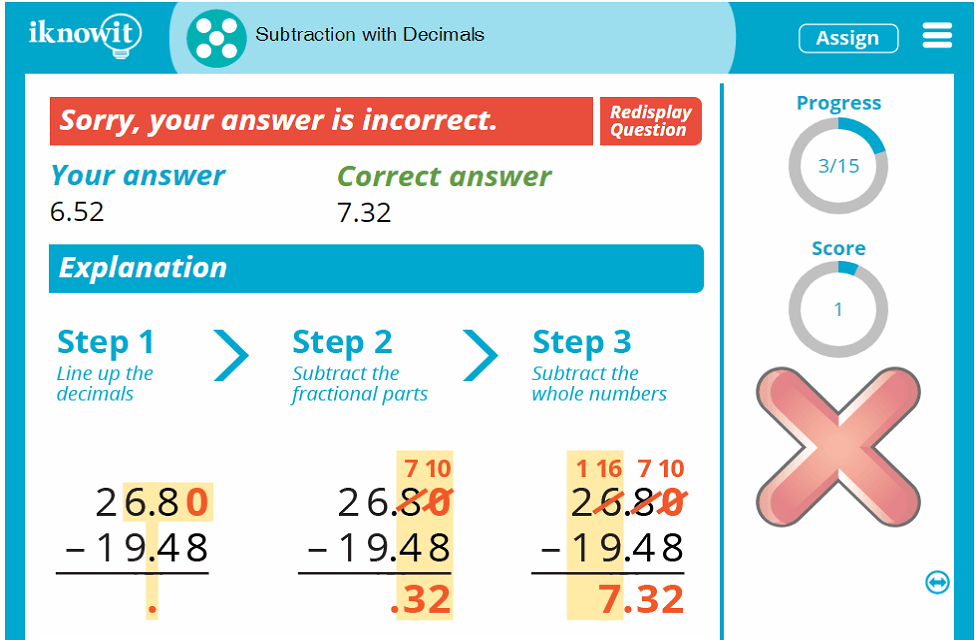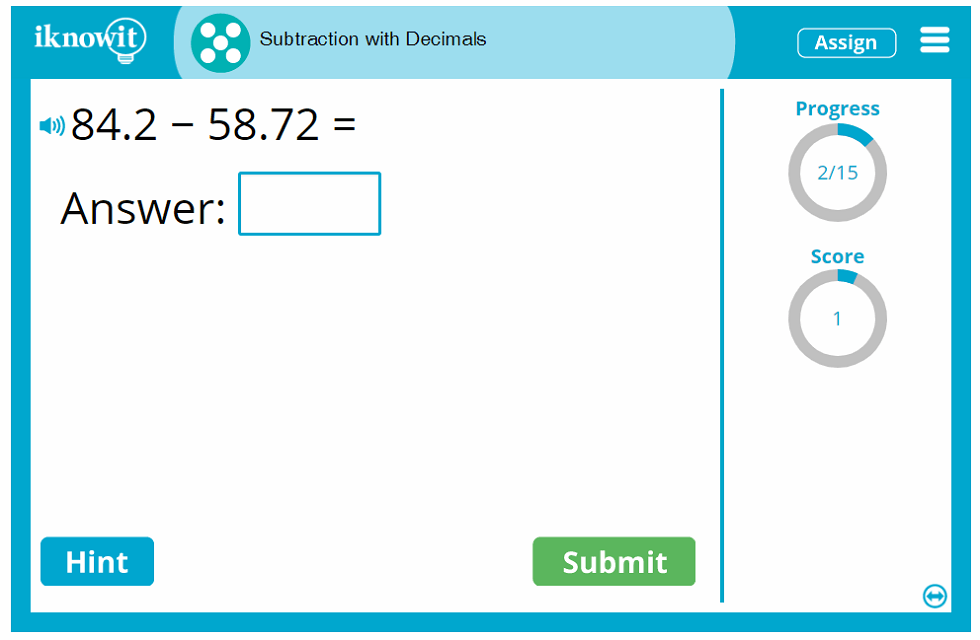## Level

This interactive math lesson is classified as Level E. It may be ideal for a fifth-grade class.

## Common Core Standard

5.NBT.B.7
Numbers and Operations in Base Ten

Students will perform operations with multi-digit whole numbers and with decimals to hundredths. Students will add, subtract, multiply, and divide decimals to hundredths.

## You might also be interested in...

In this fifth grade-level math lesson, students will practice addition with decimals. Questions are presented as word problems, vertical addition problems, and horizontal addition problems.

Addition and Subtraction with Decimals (Level E)
In this math lesson geared toward fifth grade, students will practice addition and subtraction with decimals. Questions are presented as word problems, vertical math problems, and horizontal math problems.